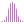#LinRegPoint (MDX)

Calculates the linear regression of a set and returns the value of y in the regression line, y = ax + b.

#### Syntax

LinRegPoint(, <List> , , )